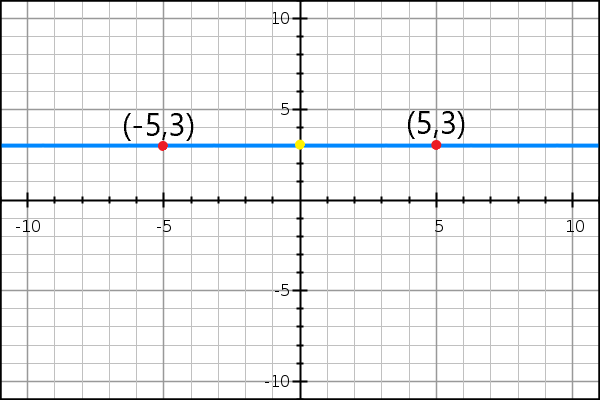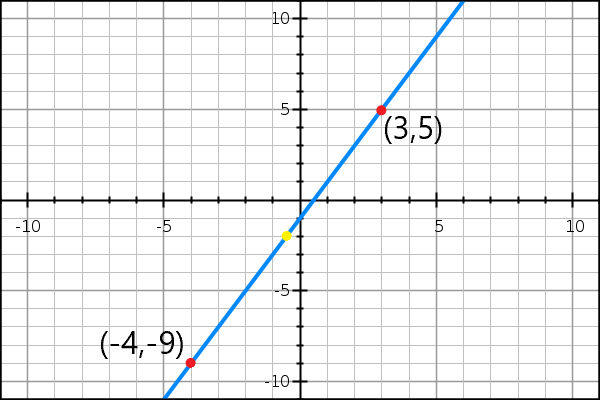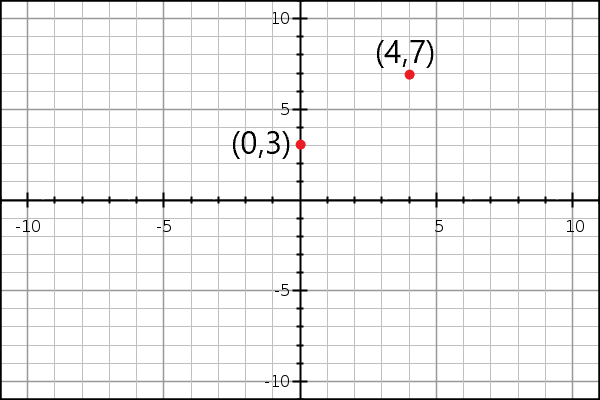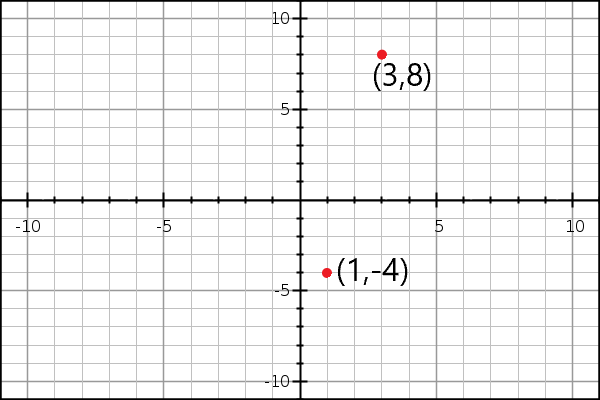# Using the Midpoint Formula

### Introduction

What is the midpoint formula? It's an algebraic tool to find the point halfway between two other points. If you imagine a line segment between two points, the midpoint lies exactly halfway along the line, like so:Geometrically, you would say that the yellow dot above is equidistant from both red points and lies on the line connecting those two points.

So, what IS the midpoint formula? Here you go:

$$(\frac{x_1+x_2}{2}, \frac{y_1+y_2}{2})$$

What does it tell you? Imagine you have two points. $$x_1$$ represents the x-coordinate of the first point, and $$x_2$$ represents the x-coordinate of the second point. Since you're dividing by 2, you're really just taking the average. The same applies for the y-coordinates.

### So, wait -- I'm just taking the average?

Yup! The midpoint between two points is just the average of the x-coordinates and the average of the y-coordinates. Let's look at a few more examples to illustrate that point. For example, in the graph below, the yellow dot is again located at the midpoint between two red points. Those points are located at (3,5) and (-4,-9). Their midpoint is located at (-.5, -2):Let's look at a quick example algebraically if you're not clear yet:

### Example 1:

Find the point halfway between $$(0,3)$$ and $$(4,7)$$:### Remember the midpoint formula:

$$(\frac{x_1+x_2}{2}, \frac{y_1+y_2}{2})$$

Plug in the appropriate values:

$$(\frac{0+4}{2}, \frac{3+7}{2})$$

$$(2, 5)$$